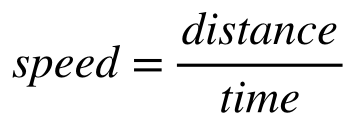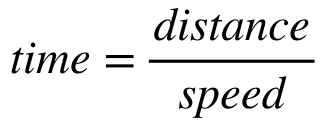# Problem: The distance from the sun to Earth is 1.496×108 km. How long does it take light to travel from the sun to Earth?

###### FREE Expert Solution

Recall that the speed of light is 3.00 x 108 m/s. Speed, distance, and time are related by:Rearranging this equation to solve for time gives us:85% (204 ratings)###### Problem Details

The distance from the sun to Earth is 1.496×108 km. How long does it take light to travel from the sun to Earth?